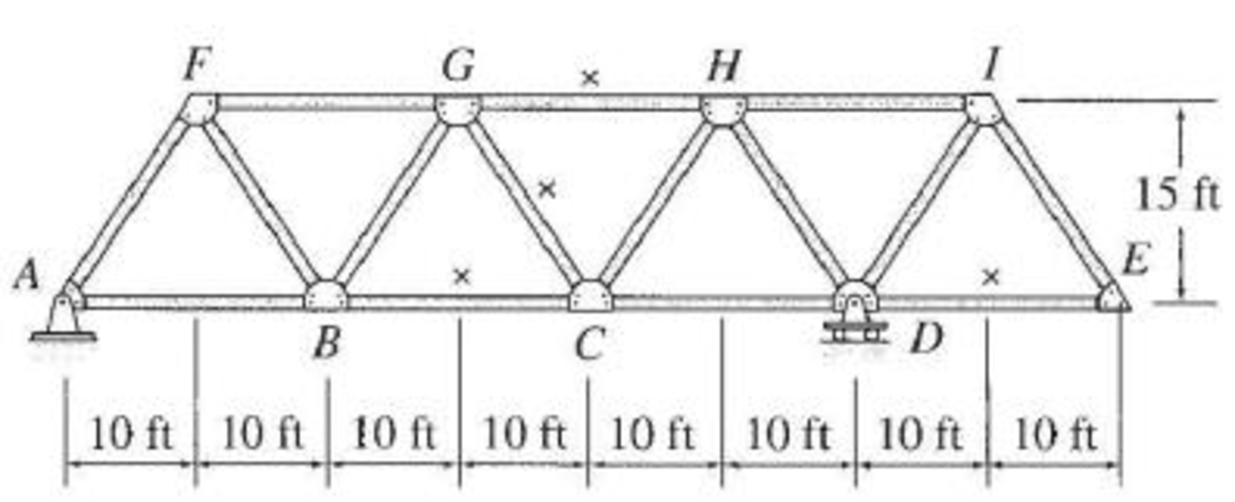# Draw the influence lines for the forces in the members identified by an “×” of the trusses shown in Figs. P8.45–P8.52. Live loads are transmitted to the bottom chords of the trusses. FIG. P8.49

#### Solutions

Chapter
Section
Chapter 8, Problem 49P
Textbook Problem
56 views

## Draw the influence lines for the forces in the members identified by an “×” of the trusses shown in Figs. P8.45–P8.52. Live loads are transmitted to the bottom chords of the trusses.FIG. P8.49

To determine

Draw the influence lines for the force in member DE, CG, GH and BC.

### Explanation of Solution

Calculation:

Influence line for the force in member DE.

Find the influence ordinate for the force in member DE at E.

The influence line for the force in member DE is determined by place a 1 k load at E.

Find the force in the member DE

The expressions for the member force FDE can be determined by passing an imaginary section aa through the members EI and DE and then apply a moment equilibrium at I.

Refer Figure 1.

Apply a 1 k load at I.

Apply moment equilibrium equation at I.

ΣMI=0

Take moment at I from E.

Consider clockwise moment as positive and counterclockwise moment as negative.

FDE(15)+1(10)=015FDE=10FDE=0.667k

Similarly find the force in member DE and summarize the value in Table 1.

 x (ft) Apply 1 k load Influence line ordinate for the force in member DE (k/k) 0 E ‑0.667 20 D 0 40 C 0 60 B 0 80 A 0

Sketch the influence line diagram for ordinate for the force in member DE using Table 2 as shown in Figure 2.

Influence line for the force in member CG.

Find the force in member CG.

The expressions for the member force FBC can be determined by passing an imaginary section aa through the members GH, CG, and BC and then apply a moment equilibrium at G.

Draw the free body diagram as shown in Figure 3.

Refer Figure 3.

Apply a 1 k load just the left of G (0x30ft).

Apply force equilibrium along vertical in the right hand section.

ΣFy=0

Consider upward force as positive and downward force is negative.

FCG(313)+Dy=0FCG=133Dy

Apply a 1 k load just the right of G (30ftx80ft).

Apply force equilibrium along vertical in the left hand section.

ΣFy=0

Consider upward force as positive and downward force is negative.

FCG(313)+Ay=0FCG=133Ay

The equation of force in the member CG,

FCG=133Dy, 0x30ft (1)

FCG=133Ay, 30ftx80ft (2)

Find the force in member CG using the Equation (1), and (2), and summarize the value in Table 2.

 x (ft) Apply 1 k load Support reaction (Ay) at A (k) Support reaction (Dy) at D (k) Influence line ordinate for the force in member CD (k/k) 0 A 1 0 0 20 B 23 13 −133Dy=−133(13)=−0.401 40 C 13 23 133Ay=133(13)=0.401 60 D 0 1 0 80 E −13 431 133Ay=133(−13)=−0.401

Sketch the influence line diagram for ordinate for the force in member CG using Table 2 as shown in Figure 4.

Influence line for the force in member GH.

Refer Figure 3.

Apply a 1 k load just the left of C (0x40ft).

Apply moment equilibrium equation at C.

ΣMC=0

Take moment at C from E.

Consider clockwise moment as negative and counterclockwise moment as positive.

Dy(20)+FGH(15)=015FGH=20DyFGH=43Dy

Apply a 1 k load just the right of C (40ftx80ft)

### Still sussing out bartleby?

Check out a sample textbook solution.

See a sample solution

#### The Solution to Your Study Problems

Bartleby provides explanations to thousands of textbook problems written by our experts, many with advanced degrees!

Get Started

Find more solutions based on key concepts
Calculate the arc length S1 in the accompanying figure. R2 =8 cm, R1 = 5 cm, and S2 = 6.28 cm.

Engineering Fundamentals: An Introduction to Engineering (MindTap Course List)

WHat should be worn when working with any type of cleaning solvent or chemical?

Automotive Technology: A Systems Approach (MindTap Course List)

What is game theory? Identify two applications of game theory.

Principles of Information Systems (MindTap Course List)

Define the terms, backup and restore.

Enhanced Discovering Computers 2017 (Shelly Cashman Series) (MindTap Course List)

What is a journeyperson?

Precision Machining Technology (MindTap Course List)

What s the Hawthorne Effect? Have you ever experienced it? When and where?

Systems Analysis and Design (Shelly Cashman Series) (MindTap Course List)

If your motherboard supports ECC DDR3 memory, can you substitute non-ECC DDR3 memory?

A+ Guide to Hardware (Standalone Book) (MindTap Course List)